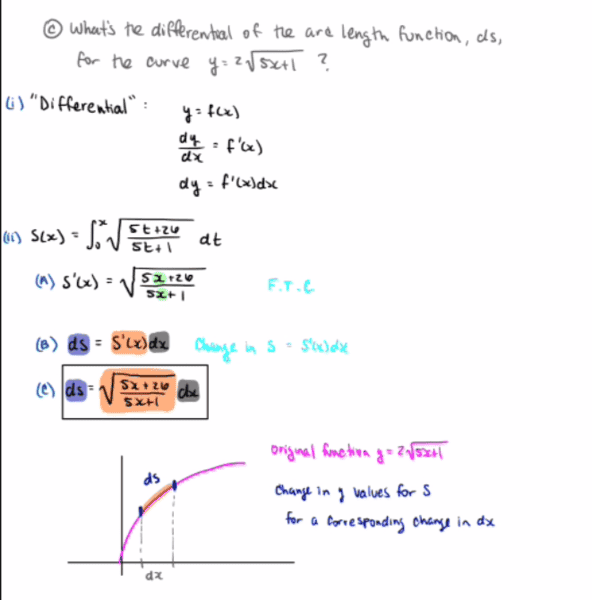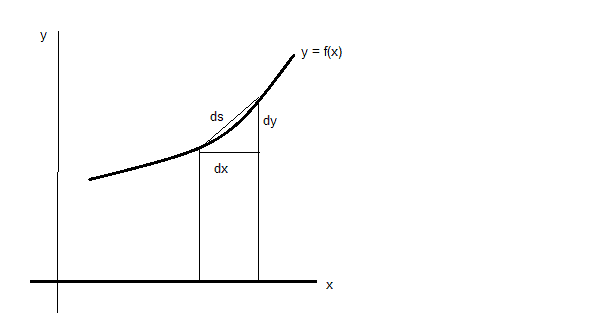# Looking for intuition on differentials and arc length

• I
Gold Member
Please see the attached image. It's a part of a study guide for my final, but I didn't put it in the homework section because I already got the answer, I just don't know what it means.
The question has to do with the differential of an arc length. I made some drawings to see if I could make some sense out of it but I don't think I'm quite there.

So when we're talking about the differential of an arc length, what exactly does this mean?#### Attachments

Homework Helper
Gold Member
##d\vec s=dx~\hat i+dy~\hat j##
##ds =\sqrt{d\vec s \cdot d\vec s}=\sqrt{(dx)^2+(dy)^2}=dx\sqrt{1+(\frac{dy}{dx})^2}##
What's ##(\frac{dy}{dx})^2~?##

•opus
Gold Member
Apologies, but I don't understand the notation. This is for Calculus I if that gives an idea. It does look like you're doing something with Pythagorean's though!

Homework Helper
Gold Member
Sorry, I thought you understood vectors. Yes, it has to do with the Pythagorean theorem. A two dimensional displacement ##ds## has a horizontal part ##dx## and a vertical part ##dy##. The two form a right triangle and ##ds=\sqrt{(dx)^2+(dy)^2}## is the hypotenuse.

Gold Member
Ok that's much more clear, thanks!
So then, since our ##dx## is extremely small, we can pretty much say that the curve of the graph is a straight line in that ##dx##.
This would give us a right triangle with the base as ##dx##, the hypotenuse as ##ds##, and the side length as what? It would have to be the change in y values corresponding the the change in x values (##dx##), but in terms of notation, what would this be?

Homework Helper
Gold Member
Look at post #2. If ##ds =dx\sqrt{1+(\frac{dy}{dx})^2}##, what is ##ds## when ##y=2\sqrt{5x+1}?##

Gold Member
##ds=dx\sqrt{\frac{5x+26}{5x+1}}##?

Homework Helper
Gold Member
Right. Does this answer your original question or is there more to it?

Gold Member
I guess I don't understand what the question is really asking me, so I suppose there's more to it. In words, how would you ask the given question to me? For example, "here is a function, what is it doing from here to here?"

Homework Helper
Gold Member
The question is asking you to find ##ds## if ##y=2\sqrt{5x+1}## and you have done that. How would you approach the following problem:
You drive your car from x = 10 units to x = 40 units along the curve given by ##y=2\sqrt{5x+1}##. How much distance has been added to your car's odometer?

Gold Member
The distance added to the odometer would be equal to the length of the curve, which is Arc Length ##= \int_{10}^{40} \sqrt{\frac{5x+26}{5x+1}}dx## which is a specific integral to the more general ##s(x)## or ##ds## too. I guess this notation is throwing me for barrel rolls. It keeps changing and I think it's distracting me from the picture.

Homework Helper
Gold Member
The distance added to the odometer would be equal to the length of the curve, which is Arc Length ##= \int_{10}^{40} \sqrt{\frac{5x+26}{5x+1}}dx## which is a specific integral to the more general ##s(x)## or ##ds## too. I guess this notation is throwing me for barrel rolls. It keeps changing and I think it's distracting me from the picture.
It's standard notation, it's part of "the picture" and eventually you will get used to it (I hope).

Gold Member
So then ##ds## is saying the change in the function ##s(x)## which is a function that gives the arc length of the function ##y##. Why are we putting it in terms of ##ds##? Why are we concerned with change at all when we are looking for the length of a curve? We had a perfectly good way to find the length when we had the formula ##s(x) = \int_{0}^{x}\sqrt{\frac{5t+26}{5t+1}}dt## What's the difference?

So then ##ds## is saying the change in the function ##s(x)## which is a function that gives the arc length of the function ##y##. Why are we putting it in terms of ##ds##? Why are we concerned with change at all when we are looking for the length of a curve? We had a perfectly good way to find the length when we had the formula ##s(x) = \int_{0}^{x}\sqrt{\frac{5t+26}{5t+1}}dt## What's the difference?
When you get to differential geometry, several basic formulas are expressed in terms of ds.

Also, check out https://en.wikipedia.org/wiki/Arc_length.

Homework Helper
Gold Member
So then ##ds## is saying the change in the function ##s(x)## which is a function that gives the arc length of the function ##y##. Why are we putting it in terms of ##ds##? Why are we concerned with change at all when we are looking for the length of a curve? We had a perfectly good way to find the length when we had the formula ##s(x) = \int_{0}^{x}\sqrt{\frac{5t+26}{5t+1}}dt## What's the difference?
Suppose someone asked you to find the length of the curve represented by the polynomial ##y=x^4+3x^3+6x## from ##x=0## to ##x=8##. What then? No formula is given to you and clearly you can't use ##s(x) = \int_{0}^{x}\sqrt{\frac{5t+26}{5t+1}}dt##. This example shows how to derive and apply the appropriate formula.

Mentor
This is for Calculus I if that gives an idea. It does look like you're doing something with Pythagorean's though!
Exactly.
So then ##ds## is saying the change in the function ##s(x)## which is a function that gives the arc length of the function ##y##. Why are we putting it in terms of ##ds##? Why are we concerned with change at all when we are looking for the length of a curve? We had a perfectly good way to find the length when we had the formula ##s(x) = \int_{0}^{x}\sqrt{\frac{5t+26}{5t+1}}dt## What's the difference?
ds represents an infinitesimal change in the length along the curve. This length depends on both dx and dy, not just dx.
The formula for s(x) that you show is not a general formula for arc length. This integral comes from the general formula for arc length, in which ds is defined as the hypotenuse on a right triangle whose legs are dx and dy.

Here's a picture.So ##ds^2 = dx^s + dy^2##, or ##ds = \sqrt{dx^s + dy^2}##. This latter equation can take different forms, using algebraic manipulations. One form is ##ds = \sqrt{1 + \left(\frac{dy}{dx}\right)^2}~dx##, if y is given as a function of x. Another form has ##1 + \left( \frac{dx}{dy}\right)^2## inside the radical, for when x is given as a function of y.

Last edited: